# DAV Class 6 Maths Chapter 2 Worksheet 7 Solutions

The DAV Class 6 Maths Book Solutions Pdf and DAV Class 6 Maths Chapter 2 Worksheet 7 Solutions of Factors and Multiples offer comprehensive answers to textbook questions.

## DAV Class 6 Maths Ch 2 WS 7 Solutions

Question 1.
For each of the following pairs of numbers, verify that product of numbers is equal to the product of HCF and LCM.
(a) 10, 15
(b) 35, 40
(c) 32, 48
Solution:
(a) 10 and 15
Product of numbers = 10 × 15 = 150
LCM of 10 and 15LCM = 2 × 3 × 5 = 30
HCF of 10 and 15HCF = 5
∴ Product of LCM and HCF = 30 × 5 = 150
Hence, product of the numbers = LCM × HCF
150 = 150
Hence verified.(b) 35 and 40
Product of numbers = 35 × 40 = 1400
LCM of 35 and 40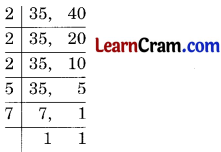LCM = 2 × 2 × 2 × 5 × 7 = 280
HCF of 35 and 40HCF = 5
∴ Product of LCM and HCF = 280 × 5 = 1400
Hence, product of numbers = LCM × HCF
1400 = 1400
Hence verified.

(c) 32 and 48
Product of numbers = 32 × 48 = 1536
LCM of 32 and 48LCM = 2 × 2 × 2 × 2 × 2 × 3 = 96
HCF of 32 and 48HCF = 16
Product of LCM and HCF = 96 × 16 = 1536
Hence, product of numbers = LCM × HCF
1536 = 1536
Hence verified.Question 2.
Find HCF and LCM by using the property of their product
(a) 27, 90
(b) 145, 232
(c) 117, 221
Solution:
(a) HCF of 27 and 90HCF = 9
LCM × HCF = Product of numbers
LCM × 9 = 27 × 90
LCM =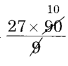= 270
Hence, LCM = 270
and HCF = 9

(b) HCF of 145 and 232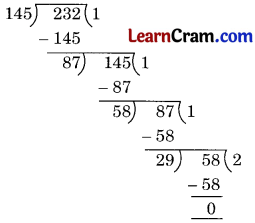HCF = 29
LCM × HCF = Product of the numbers
LCM × 29 = 145 × 232
LCM == 1160
Hence, LCM = 1160
and HCF = 29

(c) HCF of 117 and 221HCF = 13
LCM × HCF = Product of the numbers
LCM × 13 = 117 × 221
LCM = 221
Hence, LCM = 1989
and HCF = 13Question 3.
Can two numbers have 16 as HCF and 380 as LCM? Give reason.
Solution: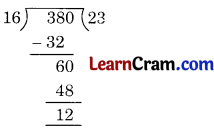No, because 16 is not a factor of 380.

Question 4.
The HCF of two numbers is 16 and product of the numbers is 3072. Find their LCM.
Solution:
LCM × HCF = Product of the numbersLCM × 16 = 3072
LCM = $$\frac{3072}{16}$$ = 192
Hence, the required LCM = 192

Question 5.
The LCM and HCF of two numbers are 180 and 6 respectively. If one of the numbers is 30, find the other.
Solution:
LCM × HCF = Product of the numbers
180 × 6 = 30 × other number 6
other number == 36
Hence, the required number = 36.Question 6.
LCM of two numbers is 1760. The numbers are 160, 352. Find their HCF.
Solution:
LCM × HCF = Product of the numbers
1760 × HCF = 160 × 352
HCF =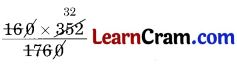= 32
Hence, the required HCF = 32.

Question 7.
Write ‘True’ or ‘False’ for the following statements:

(a) LCM of two numbers is a factor of their HCF.
Solution:
False

(b) Product of three numbers is equal to the product of their LCM and HCF.
Solution:
False

(c) HCF of given numbers is always a factor of their LCM.
Solution:
True

(d) LCM of given numbers cannot be smaller than the numbers.
Solution:
True

(e) LCM of co-prime numbers is equal to their product.
Solution:
True### DAV Class 6 Maths Chapter 2 Value Based Questions

Question 1.
The schools nowadays have a council which consists of student representatives. This council helps the school in organising various events. Rohan has also been selected in his school council this year.

The school organised a picnic in which 108, 162 and 270 students of Classes VI, VII and VIII respectively were going. Rohan’s teacher asked him to help the transport incharge.
(a) Find out the number of buses required, if each bus had to carry maximum but equal number of students from each class.
(b) As a member of school council Rohan was made a part of decision making. What other values does the student council develop in a child?
(c) Suggest one way by which you can help your school if you are selected as a council member.
Solution:
(a) Here, we have three numbers, 108, 162 and 270.
To find the maximum number of students in each bus, we have to obtain HCF of the three numbers.
We select any two numbers says 108 and 162 first.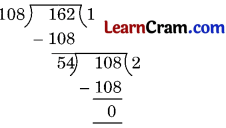So, HCF of 108 and 162 is 54.
Now, let us find the HCF of 54 and the third number, i.e., 270So, 54 buses are required.

(b) Leadership qualities, helping the needy.
(c) To maintain discipline.

Question 2.
You know 5 June is celebrated every year as World Environment Day. As a part of its celebration, Vrinda and her two friends decided to have a cycling race to promote environmental friendly transport. They started at 12 noon and took 3 minutes 20 seconds, 3 minutes 40 seconds and 4 minutes respectively to cycle on a circular track.
(a) If Vrinda and her friends started together at 12 noon, then when will they meet next?
(b) Suggest the ways by which you can save the environment.
Solution:
(a) To find when will the three friends meet next, we have to find their LCM.
It is given that Vrinda took time to cycle a circular track = 3 min 20 sec = 200 sec
First friend took time to cycle a circular track = 3 min 40 sec = 220 sec
Second friend took time to cycle a circular track = 4 min = 240 sec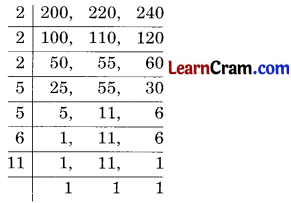LCM = 2 × 2 × 2 × 5 × 5 × 6 × 11
= 13200 sec
= 3 hr min
As Vrinda and her friends started together at 12 noon, i.e., 12:00, so they will meet again at 3:40 pm.
(b) Carpool, using CNG vehicles, etc.